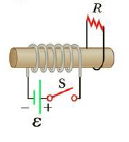Chapter 20, Problem 13P

Chapter
Section
Textbook Problem

A technician wearing a circular metal band on his wrist moves his hand into a uniform magnetic field of magnitude 2.5 T in a lime of 0.18 s. If the diameter of the band is 6.5 cm and the field is at an angle of 45° with the plane of the metal hand while the hand is in the field, find the magnitude of the average emf induced in the band.To determine
Magnitude of average induced emf in the band

Explanation

Given Info: change in magnetic field is 2.5 T, radius of the loop is 6.5×102m , orientation of the magnetic field θ=45° , and change in time is 0.18 s.

Formula for induced emf within the circle,

ε=ΔϕBΔt

• ΔϕB is the change in magnetic flux
• Δt is the change in time

Formula to calculate the magnetic flux is,

ϕBΔt=A(ΔBΔt)

• ΔB is the magnetic field,
• A is the change in area of the loop

Simplify the above expression,

ε=ΔBAcosθΔt=A(B2B1)Δtcosθ=π

Still sussing out bartleby?

Check out a sample textbook solution.

See a sample solution

The Solution to Your Study Problems

Bartleby provides explanations to thousands of textbook problems written by our experts, many with advanced degrees!

Get Started

Find more solutions based on key concepts